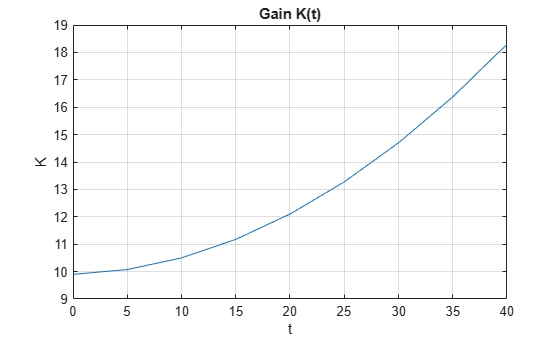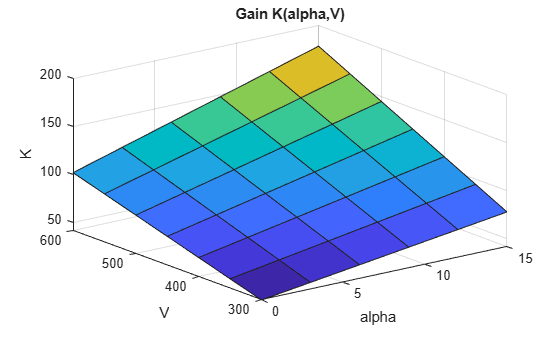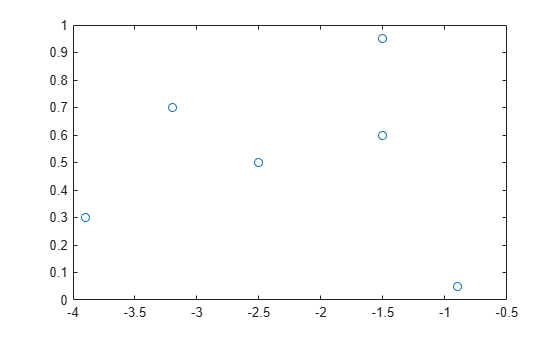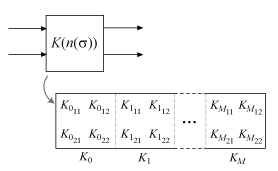# tunableSurface

Create tunable gain surface for gain scheduling

## Syntax

``K = tunableSurface(name,K0init,domain,shapefcn)``
``K = tunableSurface(name,K0init,domain)``

## Description

`tunableSurface` lets you parameterize and tune gain schedules, which are gains that vary as a function of one or more scheduling variables.

For tuning purposes, it is convenient to parameterize a variable gain as a smooth gain surface of the form:

`$K\left(n\left(\sigma \right)\right)=\gamma \left[{K}_{0}+{K}_{1}{F}_{1}\left(n\left(\sigma \right)\right)+\dots +{K}_{M}{F}_{M}\left(n\left(\sigma \right)\right)\right],$`

where

• σ is a vector of scheduling variables.

• n(σ) is a normalization function (see the `Normalization` property of the output argument `K`).

• γ is a scaling factor (see the `Normalization` property of the output argument `K`).

• F1,...,FM are user-selected basis functions.

• K0,...,KM are the coefficients to be tuned

You can use terms in a generic polynomial expansion as basis functions. Or, when the expected shape of K(σ) is known, you can use more specific functions. You can then use `systune` to tune the coefficients K0,...,KM, subject to your design requirements, over the range of scheduling-variable values.

example

````K = tunableSurface(name,K0init,domain,shapefcn)` creates the tunable gain surface: $K\left(n\left(\sigma \right)\right)=\gamma \left[{K}_{0}+{K}_{1}{F}_{1}\left(n\left(\sigma \right)\right)+\dots +{K}_{M}{F}_{M}\left(n\left(\sigma \right)\right)\right].$The tunable surface `K` stores the basis functions specified by `shapefcn` and a discrete set of σ values (the design points) given by `domain`. The tunable gain surface has tunable coefficients K0,...,KM. The gain value is initialized to the constant gain `K0init`. You can combine `K` with other static or dynamic elements to construct a closed-loop model of your gain-scheduled control system. Or, use `K` to parameterize a lookup table in an `slTuner` interface to a Simulink® model. Then, use `systune` to tune K0,...,KM so that the closed-loop system meets your design requirements at the selected design points.```
````K = tunableSurface(name,K0init,domain)` creates a flat surface with constant, tunable gain. This syntax is equivalent to `tunableGain(name,K0init)`.```

## Examples

collapse all

Create a scalar gain K that varies as a quadratic function of t:

`$K\left(t\right)={K}_{0}+{K}_{1}n\left(t\right)+{K}_{2}{\left(n\left(t\right)\right)}^{2}.$`

This gain surface can represent a gain that varies with time. The coefficients ${K}_{0}$, ${K}_{1}$, and ${K}_{2}$ are the tunable parameters of this time-varying gain. For this example, suppose that t varies from 0 to 40. In that case, the normalization function is $n\left(t\right)=\left(t-20\right)/20$.

To represent the tunable gain surface K(t) in MATLAB®, first choose a vector of t values that are the design points of your system. For example, if your design points are snapshots of a time-varying system every 5 seconds from times t = 0 to t = 40, use the following sampling grid:

```t = 0:5:40; domain = struct('t',t);```

Specify a quadratic function for the variable gain.

`shapefcn = @(x) [x,x^2];`

`shapefcn` is the handle to an anonymous vector function. Each entry in the vector gives a term in the polynomial expansion that describes the variable gain. `tunableSurface` implicitly assumes the constant function ${f}_{0}\left(t\right)=1$, so it need not be included in `shapefcn`.

Create the tunable gain surface K(t).

`K = tunableSurface('K',1,domain,shapefcn)`
```Tunable surface "K" of scalar gains with: * Scheduling variables: t * Basis functions: t,t^2 * Design points: 1x9 grid of t values * Normalization: default (from design points) ```

The display summarizes the characteristics of the gain surface, including the design points and the basis functions. Examine the properties of `K`.

`get(K)`
``` BasisFunctions: @(x)[x,x^2] Coefficients: [1x3 realp] SamplingGrid: [1x1 struct] Normalization: [1x1 struct] Name: 'K' ```

The `Coefficients` property of the tunable surface is the array of tunable coefficients, $\left[{K}_{0},{K}_{1},{K}_{2}\right]$, stored as an array-valued `realp` block.

You can now use the tunable surface in a control system model. For tuning in MATLAB, interconnect `K` with other control system elements just as you would use a Control Design Block to create a tunable control system model. For tuning in Simulink®, use `setBlockParam` to make `K` the parameterization of a tunable block in an `slTuner` interface. When you tune the model or `slTuner` interface using `systune`, the resulting model or interface contains tuned values for the coefficients ${K}_{0}$, ${K}_{1}$, and ${K}_{2}$.

After you tune the coefficients, you can view the shape of the resulting gain curve using the `viewSurf` command. For this example, instead of tuning, manually set the coefficients to non-zero values. View the resulting gain as a function of time.

```Ktuned = setData(K,[12.1,4.2,2]); viewSurf(Ktuned)````viewSurf` displays the gain as a function of the scheduling variable, for the range of scheduling-variable values specified by `domain` and stored in the `SamplingGrid` property of the gain surface.

This example shows how to model a scalar gain K with a bilinear dependence on two scheduling variables. You do so by creating a grid of design points representing the independent dependence of the two variables.

Suppose that the first variable α is an angle of incidence that ranges from 0 to 15 degrees, and the second variable V is a speed that ranges from 300 to 600 m/s. By default, the normalized variables are:

`$x=\frac{\alpha -7.5}{7.5},\phantom{\rule{1em}{0ex}}y=\frac{V-450}{150}.$`

The gain surface is modeled as:

`$K\left(\alpha ,V\right)={K}_{0}+{K}_{1}x+{K}_{2}y+{K}_{3}xy,$`

where ${K}_{0},...,{K}_{3}$ are the tunable parameters.

Create a grid of design points, (α,V), that are linearly spaced in α and V. These design points are the scheduling-variable values used for tuning the gain-surface coefficients. They must correspond to parameter values at which you have sampled the plant.

`[alpha,V] = ndgrid(0:3:15,300:50:600);`

These arrays, `alpha` and `V`, represent the independent variation of the two scheduling variables, each across its full range. Put them into a structure to define the design points for the tunable surface.

`domain = struct('alpha',alpha,'V',V);`

Create the basis functions that describe the bilinear expansion.

`shapefcn = @(x,y) [x,y,x*y]; % or use polyBasis('canonical',1,2)`

In the array returned by `shapefcn`, the basis functions are:

`$\begin{array}{c}{F}_{1}\left(x,y\right)=x\\ {F}_{2}\left(x,y\right)=y\\ {F}_{3}\left(x,y\right)=xy.\end{array}$`

Create the tunable gain surface.

`K = tunableSurface('K',1,domain,shapefcn);`

You can use the tunable surface as the parameterization for a lookup table block or a MATLAB Function block in a Simulink model. Or, use model interconnection commands to incorporate it as a tunable element in a control system modeled in MATLAB. After you tune the coefficients, you can examine the resulting gain surface using the `viewSurf` command. For this example, instead of tuning, manually set the coefficients to non-zero values and view the resulting gain.

```Ktuned = setData(K,[100,28,40,10]); viewSurf(Ktuned)````viewSurf` displays the gain surface as a function of the scheduling variables, for the ranges of values specified by `domain` and stored in the `SamplingGrid` property of the gain surface.

Create a gain surface using design points that do not form a regular grid in the operating domain. The gain surface varies as a bilinear function of normalized scheduling variables ${\alpha }_{N}$ and ${\beta }_{N}$:

`$K\left({\alpha }_{N},{\beta }_{N}\right)={K}_{0}+{K}_{1}{\alpha }_{N}+{K}_{2}{\beta }_{N}+{K}_{3}{\alpha }_{N}{\beta }_{N}.$`

Suppose that the values of interest of the scheduling variables are the following $\left(\alpha ,\beta \right)$ pairs.

`$\left(\alpha ,\beta \right)=\left\{\begin{array}{l}\left(-0.9,0.05\right)\\ \left(-1.5,0.6\right)\\ \left(-1.5,0.95\right)\\ \left(-2.5,0.5\right)\\ \left(-3.2,0.7\right)\\ \left(-3.9,0.3\right)\end{array}.$`

Specify the $\left(\alpha ,\beta \right)$ sample values as vectors.

```alpha = [-0.9;-1.5;-1.5;-2.5;-3.2;-3.9]; beta = [0.05;0.6;0.95;0.5;0.7;0.3]; domain = struct('alpha',alpha,'beta',beta);```

Instead of a regular grid of $\left(\alpha ,\beta \right)$ values, here the system is sampled at irregularly spaced points on $\left(\alpha ,\beta \right)$-space.

`plot(alpha,beta,'o')`Specify the basis functions.

`shapefcn = @(x,y) [x,y,x*y];`

Create the tunable model of the gain surface using these sampled function values.

`K = tunableSurface('K',1,domain,shapefcn)`
```Tunable surface "K" of scalar gains with: * Scheduling variables: alpha,beta * Basis functions: alpha,beta,alpha*beta * Design points: 6x1 grid of (alpha,beta) values * Normalization: default (from design points) ```

The domain is the list of six $\left(\alpha ,\beta \right)$ pairs. The normalization, by default, shifts $\alpha$ and $\beta$ so that the center of the range of each variable is zero, and scales them so that they range from -1 to 1.

`K.Normalization`
```ans = struct with fields: InputOffset: [-2.4000 0.5000] InputScaling: [1.5000 0.4500] OutputScaling: 1 ```

Create a tunable gain surface that takes two scheduling variables and returns a 3-by-3 gain matrix. Each entry in the gain matrix is an independent function of the two scheduling variables.

Create a grid of design points `(alpha,V)`.

```[alpha,V] = ndgrid(0:3:15,300:50:600); domain = struct('alpha',alpha,'V',V);```

Create the basis function that describes how the surface varies with the scheduling variables. Use a basis that describes a bilinear expansion in `alpha` and `V`.

`shapefcn = polyBasis('canonical',1,2);`

To create the tunable surface, specify the initial value of the matrix-valued gain surface. This value sets the value of the gain surface when the normalized scheduling variables are both zero. `tunableSurface` takes the dimensions of the gain surface from the initial value you specify. Thus, to create a 3-by-3 gain matrix, use a 3-by-3 initial value.

```K0init = diag([0.05 0.05 -0.05]); K0 = tunableSurface('K',K0init,domain,shapefcn)```
```Tunable surface "K" of 3x3 gain matrices with: * Scheduling variables: alpha,V * Basis functions: @(x1,x2)utFcnBasisOuterProduct(FDATA_,x1,x2) * Design points: 6x7 grid of (alpha,V) values * Normalization: default (from design points) ```

## Input Arguments

collapse all

Identifying label for the tunable gain surface, specified as a character vector. `tunableSurface` uses this name for the `realp` block that represents the tunable coefficients of the surface. Therefore, you can use this name to refer to the tunable gain coefficients within a `genss` model of a control system or an `slTuner` interface.

Initial value of the constant term in the tunable gain surface, specified as a scalar or an array. The dimensions of `K0init` determine the I/O dimensions of the gain surface. For example, if the gain surface represents a two-input, two-output gain, you can set ```K0init = ones(2)```. The remaining coefficients `K1,K2,...` always have the same size as `K0`. The tunable coefficients automatically expand so that the gains in each I/O channel are tuned independently.

For example, for a two-input, two-output surface, there is a set of expansion coefficients for each entry in the gain matrix.Each entry Kijin the tunable gain matrix K(n(σ)) is given by:

`${K}_{ij}\left(n\left(\sigma \right)\right)={K}_{i{j}_{0}}+{K}_{i{j}_{1}}{F}_{1}\left(n\left(\sigma \right)\right)+\dots +{K}_{i{j}_{M}}{F}_{M}\left(n\left(\sigma \right)\right).$`

Design points at which the gain surface is tuned, specified as a structure. The structure has fields containing the scheduling variables values at which you sample the plant for gain-scheduled tuning. For example, suppose that you want to tune a gain that varies as a function of two scheduling variables, α and V. You linearize the plant at a grid of α and V values, with α = [0.5,0.10,0.15] and V = [700,800,900,1000]

. Specify the design points as follows:

```[alpha,V] = ndgrid([0.5,0.10,0.15],[700,800,900,1000]); domain = struct('alpha',alpha,'V',V);```

The design points do not have to lie on a rectangular or regularly spaced grid (see Gain Surface Over Nonregular Grid). However, for best results use design points that cover the full range of operating conditions. Since tuning only considers these design points, the validity of the tuned gain schedule is questionable at operating conditions far from the design points.

Basis functions used to model the gain surface in terms of the scheduling variables, specified as a function handle. The function associated with the handle takes normalized values of the scheduling variables as inputs and returns a vector of basis-function values. The basis functions always operate on the normalized range [–1,1]. `tunableSurface` implicitly normalizes the scheduling variables to this interval.

For example, consider the scheduling-variable values α = [0.5,0.10,0.15] and V = [700,800,900,1000]. The following expression creates basis functions for a gain surface that is bilinear in these variables:

`shapefcn = @(x,y) [x y x*y];`

`shapefcn` is an anonymous function of two variables. The basis functions describe a parameterized gain $G\left(\alpha ,V\right)={G}_{0}+{G}_{1}{\alpha }_{N}+{G}_{2}{V}_{N}+{G}_{3}{\alpha }_{N}{V}_{N}$ where αN and VN are the normalized scheduling variables (see the `Normalization` property of `K`).

You can use anonymous functions to specify any set of basis functions that you need to describe the variable gain. Alternatively, you can use helper functions to generate basis functions automatically for commonly used expansions:

• `polyBasis` — Power series expansion and Chebyshev expansion.

• `fourierBasis` — Periodic Fourier series expansion. The basis functions generated by `fourierBasis` are periodic such that a gain surface K defined by those functions satisfies K(–1) = K(1). When you create a gain surface using `tunableSurface`, the software normalizes the scheduling-variable range that you specify with `domain` to the interval [–1,1]. Therefore, if you use periodic basis functions, then the sampled range of the corresponding scheduling variable must be exactly one period. This restriction ensures that the periodicity of the basis function matches that of the scheduling variable. For example, if the periodically varying scheduling variable is an angle that ranges from 0 to 2π, then the corresponding values in `domain` must also range from 0 to 2π.

• `ndBasis` — Build multidimensional expansions from lower-dimensional expansions. This function is useful when you want to use different basis functions for different scheduling variables.

## Output Arguments

collapse all

Tunable gain surface, returned as a `tunableSurface` object. This object has the following properties that store the coefficients, basis functions, and other information about the gain surface:

• `BasisFunctions` — Basis functions, specified as a function handle. When you create the gain surface, the `shapefcn` input argument sets the initial value of this property.

• `Coefficients` — Tunable coefficients of the gain surface, specified as an array-valued `realp` tunable parameter. The dimensions of `K0init` and the number of basis functions in `shapefcn` determine the dimensions of `K.Coefficients`.

For scalar gains, `K.Coefficients` has dimensions `[1,M+1]`, where `M` is the number of basis functions. The entries in `K.Coefficients` correspond to the tunable coefficients K0,...,KM.

For array-valued gains, each coefficient expands to the dimension of `K0init`. These expanded coefficients are concatenated horizontally in `K.Coefficients`. Therefore, for example, for a two-input, two-output gain surface, `K.Coefficients` has dimensions `[2,2(M+1)]`.Each entry Kijin the tunable gain matrix K(n(σ)) is given by:

`${K}_{ij}\left(n\left(\sigma \right)\right)={K}_{i{j}_{0}}+{K}_{i{j}_{1}}{F}_{1}\left(n\left(\sigma \right)\right)+\dots +{K}_{i{j}_{M}}{F}_{M}\left(n\left(\sigma \right)\right).$`
• `SamplingGrid` — Grid of design points, specified as a data structure. When you create the gain surface, the `domain` input argument sets the initial value of this property.

• `Normalization` — Normalization offset and scaling, specified as a structure with fields:

• `InputOffset` — Vector of offsets for each scheduling variable.

• `InputScaling` — Vector of scaling factors for each scheduling variable.

• `OutputScaling` — Scaling factor for overall gain.

In general, the `tunableSurface` parameterization takes the form:

`$K\left(\sigma \right)=\text{OutputScaling}\left[{K}_{0}+{K}_{1}{F}_{1}\left(n\left(\sigma \right)\right)+\cdots +{K}_{m}{F}_{m}\left(n\left(\sigma \right)\right)\right],$`

where n(σ) is the normalized scheduling variable, given by:

`$n\left(\sigma \right)=\frac{\sigma -\text{InputOffset}}{\text{InputScaling}}.$`

`tunableSurface` normalizes the scheduling variables to compress their numerical range and improve the numerical stability of the optimization process. By default, `OutputScaling` = 1, and `tunableSurface` computes values for `InputOffset` and `InputScaling` that map the `SamplingGrid` domain of each scheduling variable to [–1,1]. Thus, n = 0 at the center of the design-point range.

You can change the default normalization by adjusting the values of these fields. For example:

• If you have a known gain value for a particular design point, you can set `Normalization.InputOffset` so that n = 0 at that design point. You can then set `K0init` to the known gain value.

• If you want to restrict a scheduling variable to nonnegative values, set `Normalization.InputOffset` to the minimum value of that variable in your design grid. This restriction is useful, for example, when your basis function includes $\sqrt{\sigma }$.

• `Name` — Name of the gain surface, specified as a character vector. When you create the gain surface, the `name` input argument sets the initial value of this property.

## Tips

• To tune a gain surface in a control system modeled in MATLAB®: Connect the gain surface with an array of plant models corresponding to the design points in `domain`. For example, suppose `G` is such an array, and `K` represents a variable integration time. The following command builds a closed-loop model that you can tune with the `systune` command.

```C0 = tf(K,[1 0]); T0 = feedback(C0*G,1);```
• To tune a gain surface in a control system modeled in Simulink: Use the gain surface to parameterize a lookup-table, matrix interpolation, or MATLAB function block in the Simulink model. For example, suppose `ST0` is an `slTuner` interface to a Simulink model, and `GainTable` is the name of a tuned block in the interface. The following command sets the parameterization of `GainTable` to the tunable gain surface.

`ST0 = setBlockParam(ST0,'GainTable',K);`

• When you use `writeBlockValue` (Simulink Control Design) to write a tuned gain surface back to a Simulink model, the software uses `codegen` to generate MATLAB code for the gain surface. You can use `codegen` yourself to examine this code.